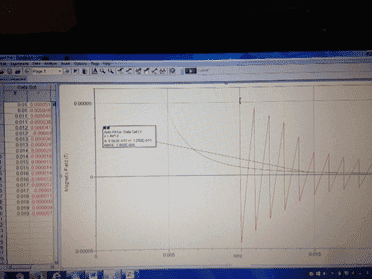# Physics Lab Permability of free space

• lloyd21

## Homework Statement

An ideal solenoid is expected to generate a dipole field that falls off quickly as you move away from the solenoid. The magnetic field at distance r along the axis of the solenoid is given by B = (Mo/ 2pie) (M/r^3) In this equation the parameter M is called the dipole moment and it is equal to M = NIA where N is the number of turns, and A
the cross-sectional area of the solenoid.
Calculate the value of the M , knowing that the number of turns in the solenoid you have is 1080 and the corss-sectional diameter is about 7.5 mm. Enter your measurements from the table above into the Logger Pro program and plot B vs r for each side of the solenoid. Then, perform a “variable power” fit of the form Y=AX^n with n set to -3 and identify the value of of the fit parameter A
Using the data you now have determine the value of the permeability of free space Mo

## Homework Equations

B= Mo I N / L

B = (Mo/ 2pie) (M/r^3)

## The Attempt at a Solution

The magnetic field at distance r along the axis of the solenoid can be determined. Breaking down the equation; N= number of turns, A= cross-sectional of solenoid area, M= dipole moment (M=NIA).

A= 7.5mm (diameter) = 0.0075m= 0.00375m (radius) =pr^2 = 4.4x10^-5m^2

Calculating the value of M; M= (1080 turns)( 4.4x10^-5m)(0.03A)

M= 1.43x10^-3 (dipole moment)

Using the data from the chart, a graph was established by inputting both sets of measurements from each side of the solenoid. A variable power fit in the form Y=Ax^n was done with n being set to -3, resulting in A = 5.56 x 10^-12 +/- 1.26x10^-11Does my graph look right? I don't know where to go from here, I've repeated this lab 3 times now and am completely stuck...Im told that ' What you want to do is to compare your fit (Y = Ax^n, with n=-3) to the theoretical relation (B = (u0/2pi)(M/r^3) ) . Comparing the two formulae can tell you what the fitted parameter "A" represents.' I am not sure how to go upon that...please help me in any way

Strange lab. The "permeability of free space" is nowadays called the magnetic constant. It is a conversion factor to connect units, not a property of vacuum. In the SI, it has the exact value of 4 pi 10^-7 N/A^2.
http://physics.nist.gov/cgi-bin/cuu/Value?mu0

I know that...that does not help for what I am looking for.

Using your reference equation, you should be able to calculate what A should be.
It seems like your fit is one or two orders of magnitude smaller than it should be.
Perhaps, you are expected to fit the peaks of your graph rather than the middle, which is clearly tending to zero much more quickly.

I calculated A, as it is the cross sectional of the solenoid.

I was referring to the other A in your post -- the coefficient of your fit curve.
## A x^{-3} \approx \frac{ \mu_0 }{2\pi}M x^{-3}##

In any case, what do you think of my recommendation to fit the peaks...or use the absolute values of your measurements, so that the oscillation doesn't force your fit down to zero so quickly?

I'm just following instructions and it doesn't say anything about fitting the peaks ...it literally just says what I posted up there...and I'm completely confused

I am suggesting that you remove the negative signs. You have negative data from one side and positive data from the other, right? They naturally are going to offset one another and force your fit curve to zero.
If you either use one side at a time, or include both sets of data, but keep the signs positive, you should get a better fit using your fit tool.